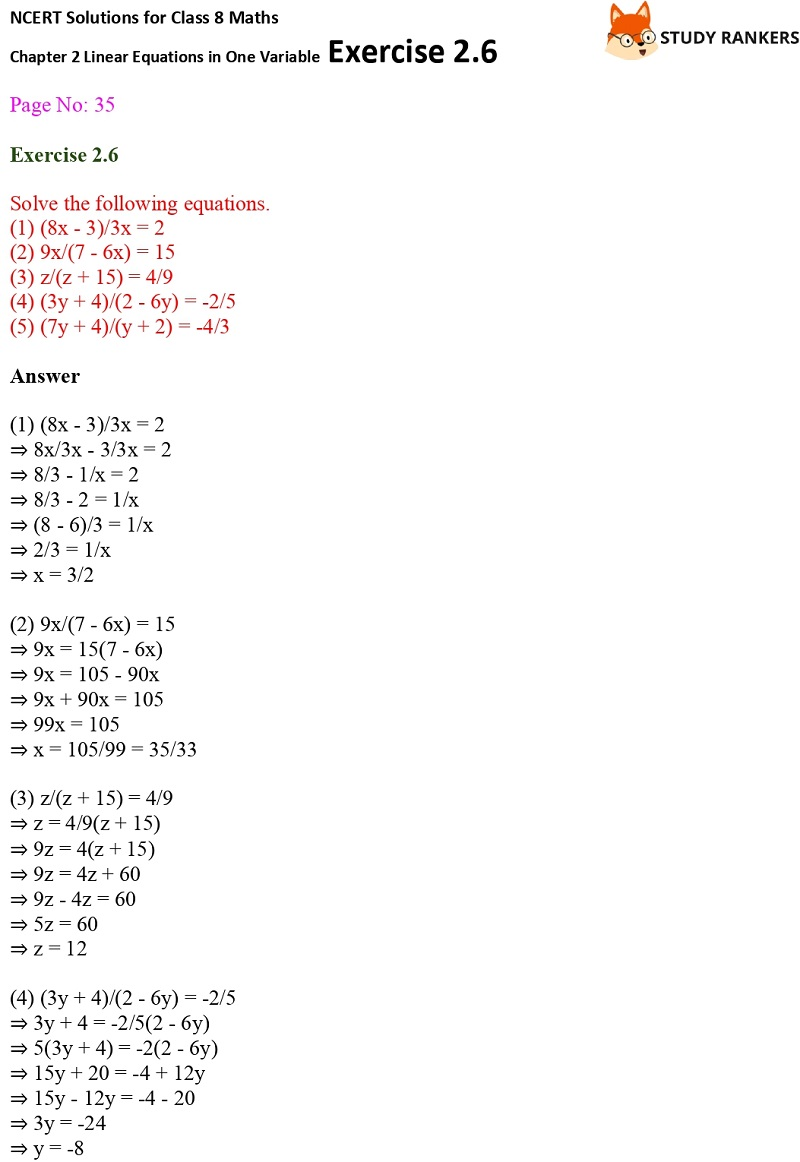# NCERT Solutions for Class 8 Maths Ch 2 Linear Equations in One Variable Exercise 2.6

NCERT Solutions for Class 8 Maths Chapter 2 Linear Equations in One Variable Exercise 2.6 present here which will be useful for improving marks in the examinations. These NCERT Solutions for Class 8 can be useful resource for inculcating correct learning habits among students.

You will find NCERT Solutions for Class 8 Maths can be find via following the link which are helpful in revising the chapter properly. In the exercise 2.6, you will find equations as well as word problems.